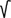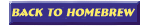Coaxial's "Z" computation

If you have a piece of unknown Coaxial cable and you are looking for the impedance ("Z") of it, probably you are in the "darkness".
In practice the solution is quite simple! If you know the inductance and the capacity of cable, then according to the following formula the impedance is:

Z=L/C

(values in microHenries (uH) & microFarads (uF)

The benefit is that, the impedance and capacity of Coaxial is independent of Coaxial's length or frequency. So, if you have for example 20m of Coaxial cable, just measure the C & L. It's enough !

Of course, you need for this job an LC-meter. Difficult sometimes, however some other Ham-friend possibly he has in his bench this istrument.

Examples:
1) I measured several meters (random length) of an unknown coaxial and i found L=1.2uH and C=450pF. Transforming the 450pF is 0.00045 uF.
(1uF = 1000000 pF, so

Z=L/C=>Z=1.2/0.00045 => Z = 51.6Ohms

2) I measured also several meters of an unknown TV coaxial and I found L=1.8uH and C=320pF. (320pF is 0.00032 uF).

Z=L/C=>Z=1.8/0.00032 => Z = 75 Ohms

The accuracy of this method is about 10%, depending upon the tolerance of LC-brigde, but in practice is high enough to determine the "Z" of unknown Coaxial cables.

Special thanks to Cliff, K7RR for the QSP of formula.

That's all folks - Have Fun
Makis SV1BSX (April 2006)